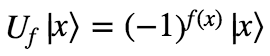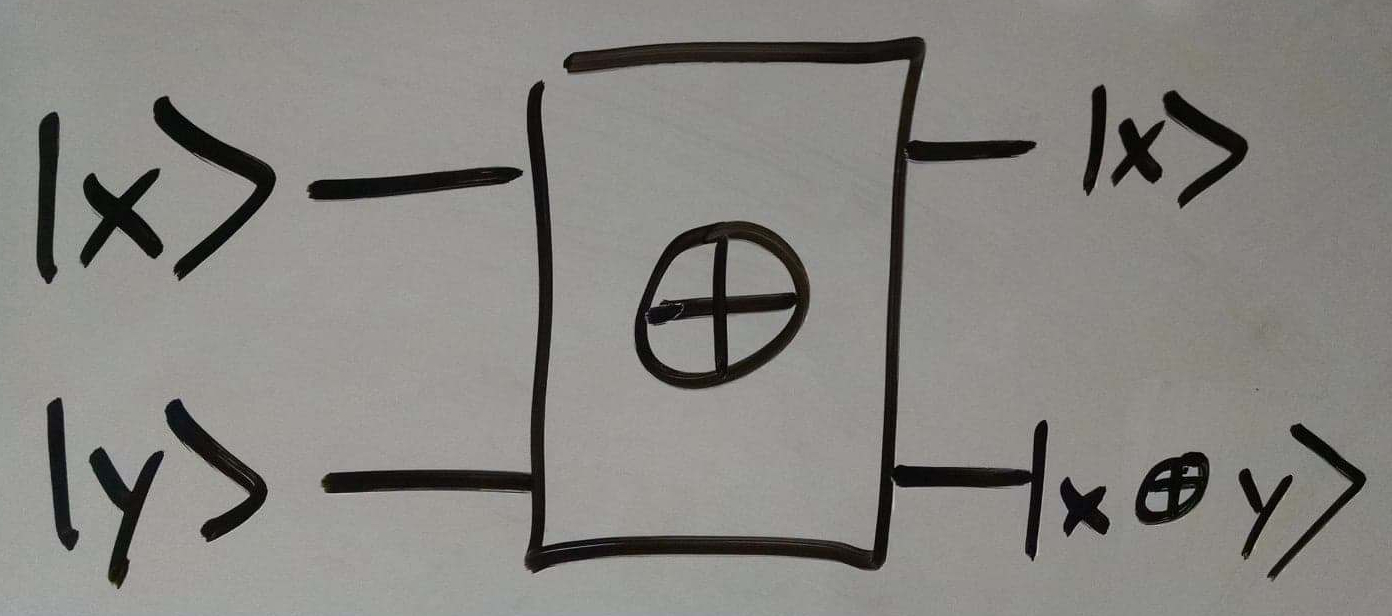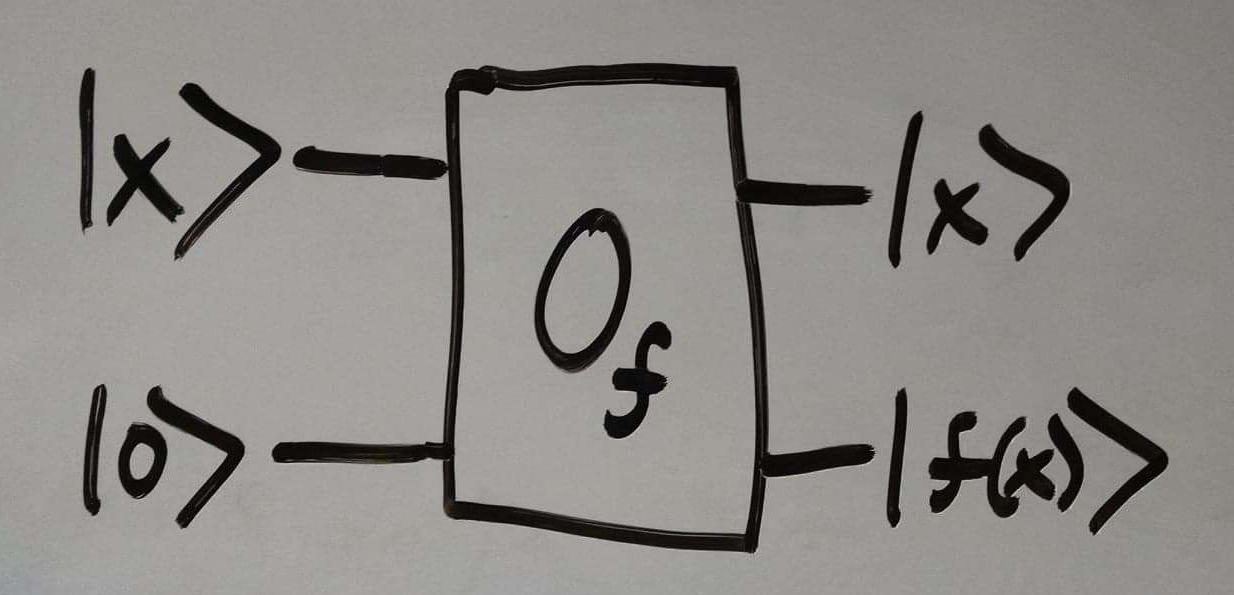# Building the quantum oracle

Part 1: Quantum Computing in a Nutshell
Part 2: More on quantum gates
Part 3: Deutch-Josza Algorithm
Part 4: Grover’s algorithm

The quantum gate Uf was featured centrally in both of the previous algorithms I presented. Remember what it does to a qubit in state |x⟩, where x ∈ {0, 1}N:I want to show here that this gate can be constructed from a simpler more intuitive version of a quantum oracle. This will also be good practice for getting a deeper intuition about how quantum gates work.

This will take three steps.

First we need to be able implement addition modulo 2 of two single qubits. This operation is defined as follows:An implementation of this operation as a quantum gate needs to return two qubits instead of just one. A simple choice might be:## 2. Oracle

Next we’ll need a straight-forward implementation of the oracle for our function f as a quantum gate. Remember that f is a function from {0, 1}N → {0, 1}. Quantum gates must have the same number of inputs and outputs, and f takes in N bits and returns only a single bit, so we have to improvise a little. A simple implementation is the following:In other words, we start with N qubits encoding the input to x, as well as a “blank” qubit that starts as |0⟩. Then we leave the first N qubits unchanged, and encode the value of f(x) in the initially blank qubit.

## 3. Flipping signs

Finally, we’ll use a clever trick. Let’s take a second look at the ⊕ gate.Then we get:Let’s consider both cases, f(x) = 0 and f(x) = 1.Also we can notice that we can get the state |y⟩ by applying a Hadamard gate to a qubit in the state |1⟩. Thus we can draw:## Putting it all together

We combine everything we learned so far in the following way:If we now ignore the last two qubits, as they were only really of interest to us for the purposes of building U, we get:And there we have it! We have built the quantum gate Uf that we used in the last two posts.